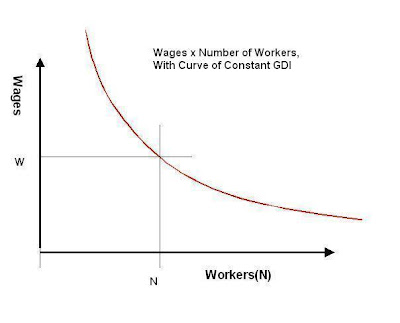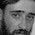## Friday, September 9, 2011

### Unemployment, Average Wage and the Distribution of Income

Employment and the Average wage.

Consider an economy where one person collected the entire national income in wages. Everyone else would be unemployed, or at least would be paid nothing.

We look at a model economy: The economy is static, that is, one whose size is some constant Y. There is only labor and labor is all paid the same, W: See: Diagram. GDI (Y) is Gross Domestic Income. Then the income of that economy is the box, any box actually, equal to Y = W x N, N the number of workers. But, this is also the productionof the economy (GDP), since everything produced will be consumed. (From the assumption of constant size, there is no change in capitalization. Capitalization changes the size of the economy. Capitalization is only a temporary expedient for consumption, since any increase in production brought about by capitalization must still eventually be consumed.)

Now, given the economy is static, what would happen if we raised (real) wages, to W’. Then the number of workers would have to decrease, to N’ where the area of the box would remain the same: W’ x N’ = Y = W x N. Unemployment would thus increase. Conversely, if we were to decrease wages to W’’, then in a static economy, the number of workers would increase, to N”, W” x N” = W x N, and unemployment decrease. (The curve of constant GDI, or Y, is a hyperbola, by the way.)Now we can consider W to be just an average wage, the mean wage, of those actually employed, (but not including those not employed,) and the arguments are the same. If we increase the mean wage, employment will decrease, if we decrease the mean wage, employment will increase. We will assign a constant: c = mean wage at full employment..

We wish to find this mean wage, (actually in general the mean of the sum of all forms of compensation,) at full employment. Given: Present total wage; Number employed at full employment. Find c.

We now go to the real world, although we treat it just like our model.

So: Total Compensation of employees received = (BEA Table 2.1) \$8264T;
Total number of workers at full employment (http://www.bls.gov/news.release/empsit.nr0.htm) =153.6M.

Then: c = \$8264T/153.6M = \$53,802, mean wage at full employment.

So we have c x N(total) = W x N, for all W and N.
Our formula for this model then would be c/W = n, where n = N/N(total) is the share of employed.

Since we are looking for unemployment rate as a function of mean wage, the variable n is equal to n = 1 - %u/100; %u equals the percentage unemployed So we substitute that expression for n: So:

w = c/(1 - %u/100)
c/w = 1- %u/100
%u = (1 - c/w) x 100

However, as a prescription, w as a function of %u is more useful. For instance, with c as defined above, = \$53,802 per capita, for a 5% unemployment rate, we would want a mean wage of \$56,634. This would be our target. Compare this to the mean wage of \$59,188 for a 9.1% unemployment rate. Some suggest that the true unemployment rate is much higher, especially if we include underemployment. An unemployment rate of 15% suggests the mean wage is at \$63,296, or nearly 12% too high for a tolerable 5% unemployment rate.

Of course this is not correct, as a higher unemployment rate than measured suggests a higher total labor force, and thus a lower c. Consider instead a total labor force of 160 Million. Then for the same values of total compensation paid employees, \$8264T, we have c = \$51,650. If we estimate 20M unemployed, for an unemployment rate of 12.5%, we have a mean wage of \$59,029, or about 9% higher than the \$54,368 mean wage required for 5% unemployment.So the prescription is to lower the mean wage to c/.95, which will result in an unemployment rate of 5%. Since this is just an average, it can be done in a number of ways, depending on the inclination of policy makers. One way would just be counting the unemployed as employed. This of course would have no real effect. A real way would be to lower every employed person’s wage by the difference. This would provide money to employ the unemployed at the same average wage. Another way we might consider would be to lower the minimum wage. Assume 20% of the labor force works at or near minimum wage. Then by lowering their wages ah, \$25,000 or so, unemployment could be reduced from 12.5 % to 5%.

Well, minimum wage is about half \$25,000. So that method is infeasible. But the same could be accomplished by lowering say the top k% in income by 100/k times the difference. Thus transferring about \$500,000 per person, on average, in the top 1%, would compensate for the difference between 12.5% unemployment and 5%unemployment. On average, since the top 1% consists of all those making more than \$400,000. But it also consists of those making much more.

The existence of this solution implies that, where there is a great disparity of income, a consequence of that disparity is a high unemployment rate.

Indeed, the calculation could be modeled on percentages: A transfer of 7.5% of national personal income from the top 1% of the income earners to the rest of the labor force would decrease the unemployment rate about the same amount, from 12.5% to 5%

The philosophy is if the boss pays himself too much, he can’t hire as many workers. Those he hires he can’t pay as well, and he can’t train them as well. Jobs that need to get done don’t, or get done badly. In today’s society, there are many jobs that need to be done, but the bosses pay themselves too much.

Note the figure we have used: Total compensation of employees received. However, we can use other figures of personal compensation: eg: \$12,091T (BEA Table 1.10 lines 2+11 Compensation of employees, paid + Net Operating Surplus, figuring the bosses also pay themselves out of corporate profits.) This merely affects c, and the absolute scale of the differences, but not the percentages.

#### 1 comment:

1.And this is the case for a progressive tax system, that removes some of the attraction for marginal greed above a certain level, in addition to obtaining a fair share from those who benefit the most from the system.